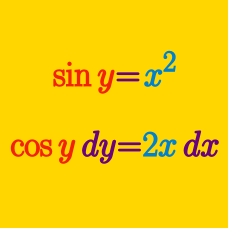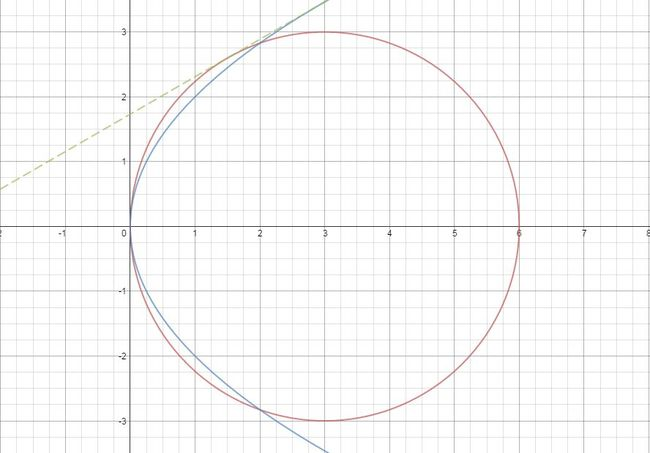Calculus

# Implicit Differentiation: Level 2 Challenges

Consider the function

$f(x) = \sqrt{x+\sqrt{x+\sqrt{x+\cdots}}}.$

If the gradient of the tangent of the function at $x = 12$ can be expressed as $\frac{a}{b},$ where $a$ and $b$ are coprime positive integers, what is the value of $a + b?$.

$\sin ( x + y ) = \log ( x + y )$

Find the value of $\frac {dy}{dx}$.

Note that we didn't give you the base of the logarithm, you should not specifically assume that the logarithm is in base $e$ or 10.

If ${ x }^{ y }={ e }^{ x-y }$, then $\frac { dy }{ dx } = \text{\_\_\_\_\_\_\_\_\_\_\_\_\_\_\_}.$Which of the given equations is the common tangent to the circle ${(x-3)}^2+{y}^2=9$ and the parabola ${y}^2=4x$ touching both the conics above the $x$-axis?

Consider the function $\displaystyle f(x) = \frac{x}{1+\frac{x}{1+\frac{x}{1+\ddots}}}.$

Determine the value of $f'(0).$

×